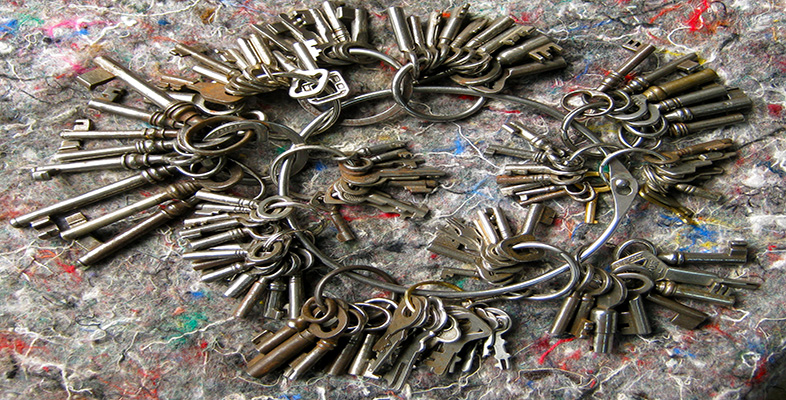Education & Development

### Become an OU studentKey skills: making a difference

Start this free course now. Just create an account and sign in. Enrol and complete the course for a free statement of participation or digital badge if available.

# 7.5.2 Title

Being able to understand and use a range of numerical, graphical, algebraic and other mathematical techniques is a central feature of number skills. Use the following list to help you identify the areas you may need to work on. Will you need to:

• make observations, measurements, and express and combine the units of measurement correctly?

• read and interpret scale drawings, graphs, tables and charts? If so, do you need to learn about the styles and conventions used to present graphical and numerical data?

• organise and classify data? If you need to use a software package to handle and present your data, you may need to schedule some time to learn how to use the software package effectively;

• make inferences and draw conclusions from sets of data by calculating statistics such as mean, standard deviation, and interquartile range? If so, can you interpret the mathematical notation used to express the relevant formula?

• use numerical, graphical and algebraic methods to develop models? You may need to find out how tables of data, graphs and formulae can be related to each other, and what the advantages and disadvantages are of each way of modelling a relationship;

• use ideas of proportion, variation and scaling, including inverse proportion and other non-linear variations? If so, do you understand the difference between a linear and a non-linear relationship?

• work with expressions, formulae and equations, including powers and roots? If so, can you rearrange a formula to express an unknown quantity in terms of known quantities?

• work with probability? You may need to find out how the probabilities of single or multiple events are calculated and interpreted;

• make deductions in algebraic and spatial reasoning and be able to manipulate symbols or shapes as part of your mathematical problem-solving technique?

In carrying out any calculations – whether manually, using a calculator, spreadsheet or other software package – you need to know how to choose the levels of accuracy appropriate to the task, and how to check for errors. Make sure you understand how errors can accumulate when you use approximate data or numbers rounded to significant figures, and what the effect will be on the overall result of your calculations.

## Time out

In your Skills File, identify the mathematical areas you need to work on, including any that are not in the above list. Note the area you feel least confident about,